# After getting acquainted with the basic concepts of information security, we want to enter the topic of cryptography and get acquainted with different methods of cryptography.

### Cryptography, In the previous article of the Proceedings of the principles of information security with the key concepts of information security were introduced.

In this article, we start the topic of cryptography, and after getting acquainted with the basic definitions in this field, we examine the simple substitution method to use it to say what is meant by a secure cryptography method.

## Remember these words

First of all, it is better to get acquainted with the definition of these words. Knowing these terms will help you to know exactly what they mean, especially if you come across them in specialized texts, and secondly, if you want to read more about these topics, you know exactly what phrases to look for.

Cryptology : Knowledge of making and breaking secret codes.

Cryptography : Knowledge of creating secret codes.

Cryptanalysis : Knowledge of breaking secret codes

You may also come across the word Crypto in specialized texts, which can mean any of the above three words. Depending on the text, you can tell which meaning is meant.

One crypto system ( Crypto System – Cipher ) receives the original data as input and returns another data as output, which is equivalent to the encrypted original data. So the original data as input to the system is encrypted plain text ( Plain text ) say and the encryption system output ciphertext ( Cipher text ) is called.

A procedure that converts plain text to ciphertext Encryption ( Encryption ) and it’s contrary to the plain text into ciphertext decryption ( Decryption ) is called. Also in the operation of a key ( Key) Is also used to configure the cryptographic system. The following figure gives a better understanding of the above definitions.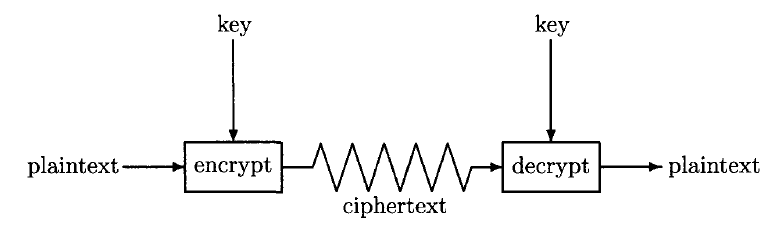#### Cryptographic methods are divided into two general categories, symmetric and asymmetric.

In symmetric encryption ( Symmetric cipher ) is a key for encryption and decryption operations.

When using this method, the key must be kept confidential because anyone who has access to the key can convert the ciphertext to plain text. Unlike the procedure in asymmetric cryptography ( Asymmetric ) of the two keys are used.

One key to encrypt information and the other key to decrypt. When this encryption method is used, the key used for decryption is usually made available to the public and the other key is kept confidential. That’s why these two keys, public key ( Public Key ) and the private keyPrivate Key ) is called.

When someone uses public key to decrypt information, they can ensure that the information is encrypted by the same person who holds the private key and is not manipulated by someone else. In this way encryption, encrypted with the public key ( Public key encryption ) is also called.

In an encryption system ideal ( Ideal Cipher ) to obtain the plaintext and ciphertext using the keyless impossible.

The security of a cryptographic system must be key-based. For added security, details of how the cryptographic system works should not be hidden. In fact, everyone should be able to have accurate information about how the cryptographic system works, and all that needs to be hidden is the key. This is known as the Kirkhoff principle , which should be considered in the design of cryptographic systems.

#### Substitution Cipher

You must remember Jafar, Morteza and Javad from the first article.Morteza wants to send a message to Jafar Bank and in this message his transaction information is written. Jawad Ibn is able to listen to the messages exchanged. For this reason, Morteza can not send the original message to Jafar Bank.

You have to provide him with an encryption system so that even if the message being exchanged is in Javad’s possession, Morteza’s account information will not be revealed.

To do this we can use a simple encryption system called substitution cipher ( Substitution Cipher ) use.

In this cryptographic system, we use another letter instead of each letter of the alphabet. In its simplest form, instead of each letter of the alphabet, we use another letter that is a certain number after that letter. And In this case, the space between the main letter and the letter that replaces it is used as the encryption key.

#### The following figure shows how to replace the letters.

In this example, instead of each letter, the letter in the alphabetical order of 3 is used, so here is the cryptographic key 3.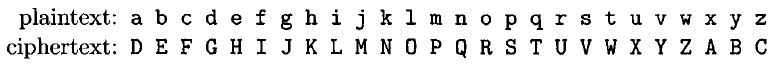This encryption system is known as Caesar encryption when used with key 3. In ancient Rome, this method was used to encrypt confidential information.

According to the figure above, if n = 3 is used as the encryption key Message: thisismorteza is encrypted as WKLVLVPRUWHCD. To decrypt it, it is enough to do the opposite. That is, instead of each letter in the encrypted message, we put the 3 letters before it in alphabetical order.

By doing this, can we be sure that if Javad receives the encrypted message, he will not be able to understand the content of the original message?

Another issue that needs to be taken as the key ( Key Space ) is. Key space means all values ​​that may be used as encryption keys. In the simple substitution cryptography method we examined, in English, given that there are 26 letters in the alphabet, the key can have values ​​from 0 to 25. As a result, the size of the key space in this method is equal to 26.

Thus, if Javad knows that simple substitution encryption has been used, in order to obtain the encryption key, he must try all 26 possible values ​​to obtain the key. It’s not difficult to try all these 26 keys. So we can not say that this encryption mechanism provides sufficient security.

This type of attack where all the switches are looking for real value encryption key is searched comprehensive search key ( Exhaustive search key ) is called.

## How big should the key space be?

We have seen that simple substitution encryption is not a good way to encrypt information,

because there is only one value that can be used as a key, and thus anyone who wants to break this encryption only needs to use these 26 values ​​as a one-to-one key. Do an experiment to find the right key.

But how big does the key space really have to be to make sure encryption can’t be attacked this way?

Suppose Javad’s computer has enough computing power to test 2.40 keys per second.

In this case,

if the number of possible keys is 2.5 x 56, it only takes 2.16 seconds, which is about 18 hours for Javad to try all possible keys and find the right key.

Similarly, if the size of the key space reaches 2.64 , it takes more than 6 months, and with a key space of ^ 128 بیش more than 90 trillion centuries, it takes Javad to try all the possible keys with his computer.

So we need to make a change to the simple replacement encryption method to make the key space large enough. To do this, we change the way letters are substituted so that each letter can replace any other letter.

This means that we can have 26 choices to choose which letter to use instead of the letter a,

and then we will have 25 choices to choose the successor to the letter b (obviously we can not choose the letter that replaces the letter a) And so to select the 26 key! That means we have about 2.88 options.

This key space is large enough that Javad can not find the cryptographic key by trying all the keys. The following figure is an example of a key in this encryption method.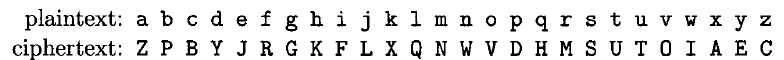## Cryptanalysis

We said that cryptanalysis or Cryptanalysis of cracking. Now we want to see with a simple example what it is like to break a cryptographic method. Suppose Javad succeeds in listening to this message: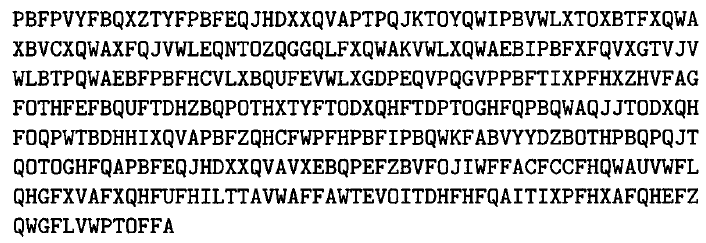Given that the cryptographic key can be any permutation of English letters, it is not possible for Javad to try all possible keys. So he has to use a smarter way to get to the content of the message.

#### The following is a graph of the frequency of letters in English.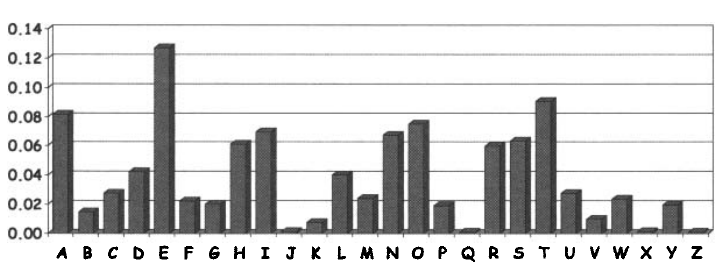And this figure is a diagram of the frequency of letters in the message that Javad overheard.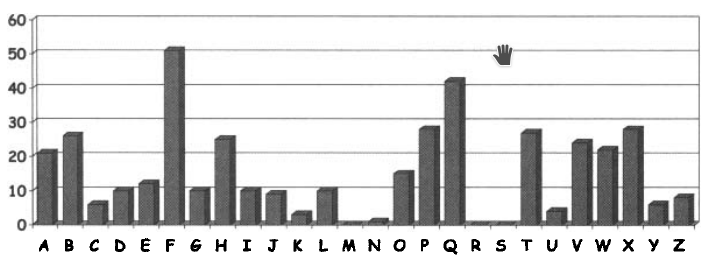Looking at these messages and diagrams, one can guess that the letter F is used in the encrypted message instead of the letter e.

The first word is usually easier to guess than any other word.

Looking at the diagrams and knowing that the third letter was actually e instead of F, one can guess that the first word in the main message was the.

With this assumption in mind, the other two letters of the message are identified and can be used to find the next letters.

Something like solving a table.

Finally,

by continuing the same method, Javad can decrypt the message or make the remaining part of the key so small that it is possible to get the right key by trial and error.

So large key space, although necessary to have a secure encryption method, is not enough. How do we ensure a cryptographic method is secure?

## What is meant by safe Cryptography?

If we can have a mathematical proof that it is not possible to get from encrypted text to plain text without a key,

we can be sure that the cryptographic method we use is secure. But such proof usually does not exist.

No such proof has been provided for any of the most widely used cryptographic methods.

In the absence of a mathematical proof of cryptographic system security,

if the best known attack on a cryptographic method is not computationally possible, we can consider that cryptographic system to be reliable.

In the series of articles where the term secure ( Secure ) encryption system used for an attack,

we mean is best known for its encryption system nearly as comprehensive search key ( Exhaustive key search ) requires time and computing power.